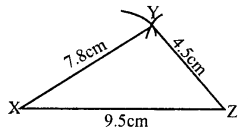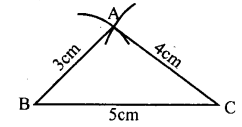# KSEEB Solutions for Class 8 Maths Chapter 12 Construction of Triangles Ex 12.1

Students can Download Maths Chapter 12 Construction of Triangles Ex 12.1 Questions and Answers, Notes Pdf, KSEEB Solutions for Class 8 Maths helps you to revise the complete Karnataka State Board Syllabus and score more marks in your examinations.

## Karnataka Board Class 8 Maths Chapter 12 Construction of Triangles Ex 12.1

Question 1.
Construct ∆ABC in which AB = 5 cm BC = 4.6 cm and AC = 3.7 cm.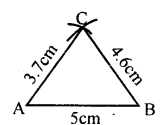Question 2.
Construct an equilateral triangle of side 4.8 cm.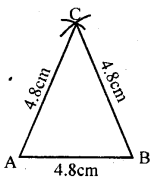Question 3.
Construct ∆PQR given PQ = 5.6 cm, PR = 7 cm and QR = 4.5 cm.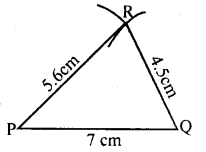Question 4.
Construct a triangle XYZ in which XY = 7.8 cm, YZ = 4.5 cm and XZ = 9.5 cm.